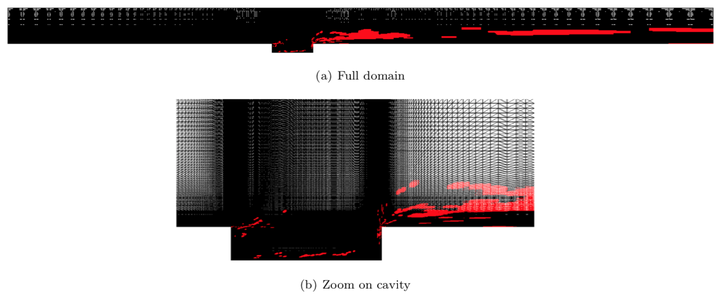# Galerkin v. least-squares Petrov--Galerkin projection in nonlinear model reductionSample mesh (red) embedded within original mesh. The sample mesh is used to to enable low-computational-footprint simulations with the GNAT reduced-order model.

### Abstract

Least-squares Petrov–Galerkin (LSPG) model-reduction techniques such as the Gauss–Newton with Approximated Tensors (GNAT) method have shown promise, as they have generated stable, accurate solutions for large-scale turbulent, compressible flow problems where standard Galerkin techniques have failed. However, there has been limited comparative analysis of the two approaches. This is due in part to difficulties arising from the fact that Galerkin techniques perform optimal projection associated with residual minimization at the time-continuous level, while LSPG techniques do so at the time-discrete level. This work provides a detailed theoretical and computational comparison of the two techniques for two common classes of time integrators: linear multistep schemes and Runge–Kutta schemes. We present a number of new findings, including conditions under which the LSPG ROM has a time-continuous represen- tation, conditions under which the two techniques are equivalent, and time-discrete error bounds for the two approaches. Perhaps most surprisingly, we demonstrate both theoretically and computationally that decreasing the time step does not necessarily decrease the error for the LSPG ROM; instead, the time step should be ‘matched’ to the spectral content of the reduced basis. In numerical experiments carried out on a turbulent compressible-flow problem with over one million unknowns, we show that increasing the time step to an intermediate value decreases both the error and the simulation time of the LSPG reduced-order model by an order of magnitude.

Publication
Journal of Computational Physics, Vol. 330, p. 693–734 (2017)